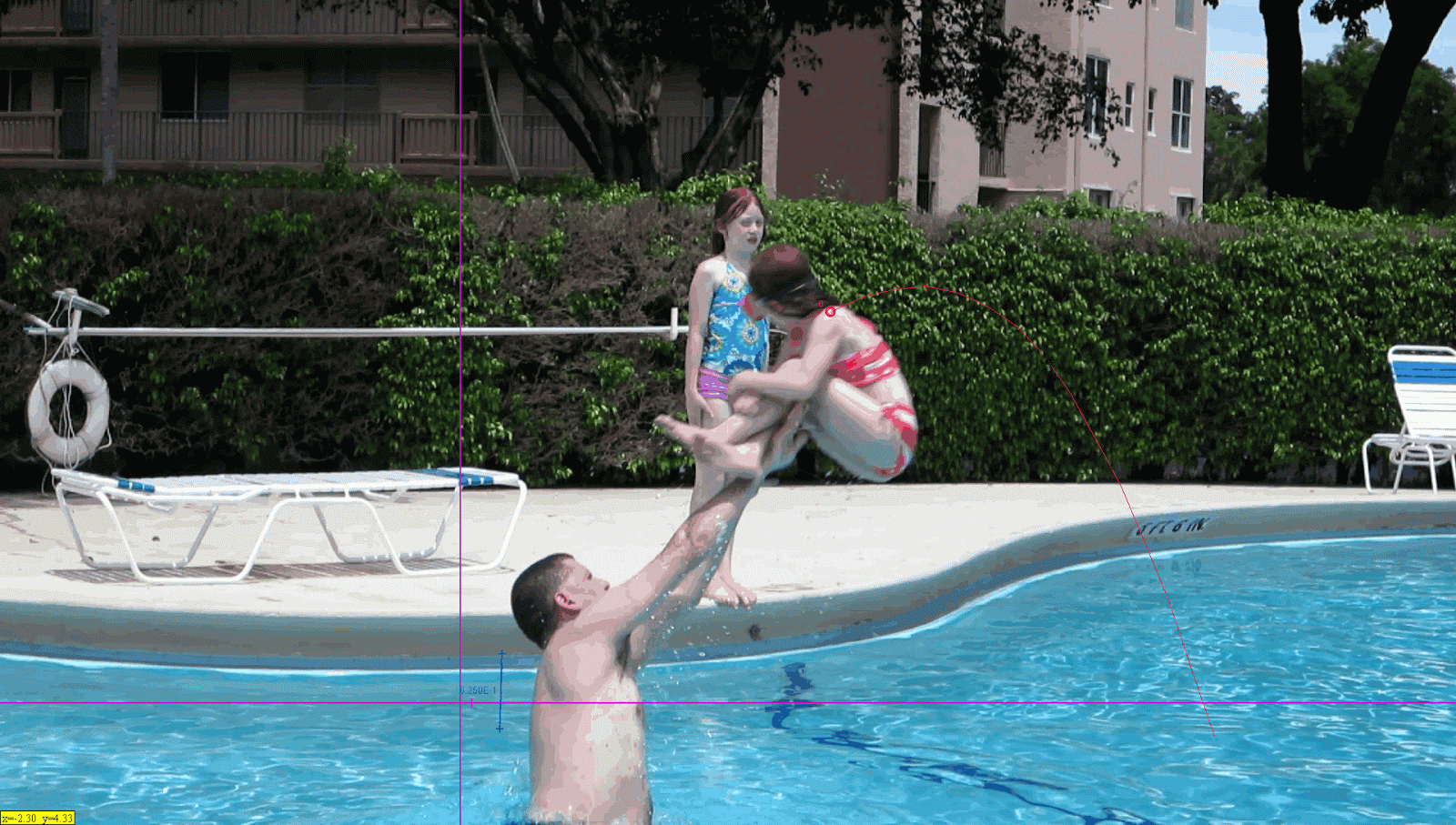# Lab 6.2 - Parabolic MotionParabolic motion is what happens when you pass a ball, fire a cannon, or launch a car into the air with a trebuchet. Or throw a child into a pool.

Create a program that will take the initial velocity in meters per second and angle in degrees of a projectile and calculate the projectile's time in flight, maximum height, and distance. Assume negligible friction and wind, and a starting height of 0 meters. Round all answers to the nearest two decimal places.

If you need some help, you might find these equations useful.

Here are some test values and answers (rounded) for you to test:

• Initial velocity 100, angle 45: range 1,020.41; max height 255.1; flight time 14.43.
• Initial velocity 23, angle 12: range 21.96; max height 1.17; flight time 0.98.
• Initial velocity 1337, angle 23: range 131,211.18; max height 13,923.96; flight time 106.61.

Use meters, seconds, and degrees for units. As always, design a useful and proper interface and use good software engineering practices.